﻿ Technique for Continuation Power Flow Using the “<i>Flat</i><i> </i><i>Start</i>” and for Ill-Conditioned Systems

### Technique for Continuation Power Flow Using the “FlatStart” and for Ill-Condit...

Bonini Neto A., Matarucco R. R., Alves D. A.OPEN ACCESSPEER-REVIEWED

## Technique for Continuation Power Flow Using the “FlatStart” and for Ill-Conditioned Systems

Bonini Neto A.1,, Matarucco R. R.2, Alves D. A.3

1Department of Biosystems Engineering, UNESP- São Paulo State University, Tupã, Brazil

2Votuporanga University Center - UNIFEV, Votuporanga, SP, Brazil

3Department of Electrical Engineering, UNESP- São Paulo State University, Ilha Solteira, Brazil

### Abstract

This paper presents a geometric parameterization technique for the continuation power flow that allows the complete tracing of P-V curves and the calculation of the maximum loading point of power systems, without singularity problems of Jacobian matrix (J). The technique will be started from the “flat start”, i.e., the voltage magnitude of all the buses PQ are placed in 1.0 p.u. and the angle bus voltage of all the buses are placed at 0º (zero degree). To eliminate the singularity has been added to the equation of a line passing through a point in the plane determined by the variable load factor and the sum of all the bus voltage magnitudes. The results obtained with the technique to ill-conditioned systems (11 buses), IEEE test systems (300 buses) and the 638-bus system corresponding to part of South-Southeast Brazilian system, improve the characteristics of the conventional method and extend the convergence region around the singularity.

### At a glance: Figures

123
Prev Next

• A., Bonini Neto, Matarucco R. R., and Alves D. A.. "Technique for Continuation Power Flow Using the “Flat Start” and for Ill-Conditioned Systems." World Journal Control Science and Engineering 3.1 (2015): 1-7.
• A., B. N. , R., M. R. , & A., A. D. (2015). Technique for Continuation Power Flow Using the “Flat Start” and for Ill-Conditioned Systems. World Journal Control Science and Engineering, 3(1), 1-7.
• A., Bonini Neto, Matarucco R. R., and Alves D. A.. "Technique for Continuation Power Flow Using the “Flat Start” and for Ill-Conditioned Systems." World Journal Control Science and Engineering 3, no. 1 (2015): 1-7.

 Import into BibTeX Import into EndNote Import into RefMan Import into RefWorks

### 1. Introduction

The data from the National System Operator (NSO)  consider as one of the Brazilian scenarios, an average rate of annual growth of Gross Domestic Product (GDP) of 5.0% for the five year period 2011-2015, which representing an annual growth load rate for the same period equivalent to same 5.0% . Forecast like this leads to the need to build new hydroelectric dam, as well as interconnections between the various subsystems. However, environmental problems, and especially in Brazil, the great distances between the load centers and hydroelectric dam and the systems presenting high compensation, make and will make the system operate close to its safety limits. These safety limits are associated with the voltage stability which is related to the lack of support of reactive power necessary to maintain a satisfactory voltage profile for the electric power system .

The conditions described in the previous paragraph are not related only to Brazil, but in general, the whole world. Thus, companies connected to sector, and research institutions (public and private universities, and others), have struggled to develop work related to voltage stability problem. These works aim to provide conditions for the steps of planning and operation of an electric power system, are defined the voltage stability margins (loading margin) and the control measures required for the normal conditions system operation (base case) and to the different conditions of contingencies that may eventually occur .

In Matarucco et al. , an alternative methodology was presented for static contingency analyses that only use continuation methods and thus provides an accurate determination of the loading margin. Rather than starting from the base case operating point, the proposed continuation power flow obtains the post-contingency loading margins starting from the maximum loading and using a bus voltage magnitude as a parameter. The branch selected for the contingency evaluation is parameterized using a scaling factor, which allows its gradual removal and assures the continuation power flow convergence for the cases where the method would diverge for the complete transmission line or transformer removal.

An electrical power system is exposed to the occurrence of a large number of contingencies. Thus, before the voltage stability analysis, is carried out the selection and ordering of contingencies according to the impact they cause to the system, thereby reducing the computational time of the analysis.

In the assessment of voltage stability where should trace P-V and Q-V curves for each contingency, static analysis methods are used, as the load flow method and continuation power flow, which must be efficient and reliable in order to meet the requirements needed for applications in planning stage and operation in real-time.

This paper presents a geometric parameterization technique which was previously initialized in , which aims to get the complete tracing of P-V curves and get the maximum loading point (MLP) and subsequently presented in this paper, assessing the voltage stability margin of ill-conditioned power system (networks whose models resulting in systems of equations difficult to solve [5, 6]) and started from the “flat start” (voltage magnitude of all the buses PQ are placed in 1.0 p.u. and the angle bus voltage of all the buses are placed at 0º (zero degree)).

### 2. Ill-Conditioned Systems and Flat Start

According to , are called ill-conditioned systems all those who, with loading within the feasible region, have no solution using the conventional load flow. This name is given both the systems close to the voltage stability limit as for systems away from this, provided that present problems of convergence during the iterative process .

In , however, the author calls attention to mathematical definition of ill-conditioning. According to , the matrix is considered ill-conditioned if your condition number is large enough. The condition number corresponds to the ratio between the largest and the smallest eigenvalues of the matrix J [8, 9]: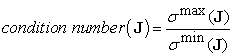(1)

In the load flow problem, the matrix of interest is the Jacobian. As a high value of condition number is associated with small singular values and/or eigenvalues, and the voltage collapse is associated with small eigenvalues, the author states that ill-conditioning is voltage stability problem.

As a priori, does not know the system to be studied and most ill-conditioned systems have loading margin lower than base case, it was decided to start the method from default values “flat start” (all voltages in load buses initialized with unitary module and zero phase angle).

### 3. Continuation Power Flow

The proposed continuation power flow consist to find a continuation power flow solution for a given load change condition.

The goal of the method is to trace the buses voltage profiles from a known initial solution (base case) and using a predictor corrector scheme find solutions subsequent to the maximum loading point. This process can be obtained voltage stability margin, and additional information about the voltage behavior of the system buses with the increased of the loading level.

In general, the power flow equations for the system can be written as: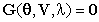(2)

where G is a vector composed by balance equations of nodal real and reactive power. The equation (2) can be written as: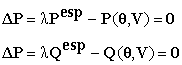(3)

where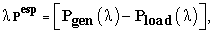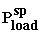,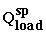andare specified values at base case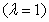for real and reactive power of PQ buses and real power for PV buses, respectively. The symbols kpg, kpl and kql are pre-specified parameters used to adjust a specific loading scenario, describing the rate of changing of real power (Pgen) for generation buses (PV buses), and real (P) and reactive (Q) power for load buses (PQ buses). Therefore, it is possible to apply an individual loading variation, for a single bus or for a selected group of buses, considering different power factors (from the base case values) for the buses.

Traditionally, however, it is assumed that the increased load of a certain area is done with a constant power factor and proportional to the loading of the base case with constant power load model, this provides the most secure operating condition for the system [10, 11].

To obtaining of P-V curves, the addition of parameterized equations in the equations system (3) has been a standard procedure . In a general way, the equation to be added can be placed in the following form:(4)

where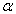andare angular coefficients that defining the line to be used, and [y] is the scalar measure of the vector y=[y1,...,yn]T, which can be chosen from various forms: 

[y]= yk where k is any of the indexes1≤ k n;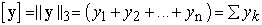(5)

The first form is among local parameterization techniques [12, 13, 14, 15, 16], whereas the second is an example of the global parameterization  and [17, 18, 19, 20].

In the method proposed in , the process starts by usingas parameter, then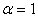and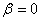and when [y] is the parameter,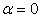and.

In this work it is considered, where y is the vector of nodal voltage magnitudes (V), i.e., sum of nodal voltage magnitudes of all buses of the system. The parameteris the angular coefficient of the line passing through a chosen point “O”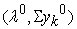in the plane formed by the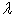andvariables. In this case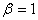was chosen. By adding this equation,can be treated as a dependent variable andis regarded as an independent variable, that is, it is chosen as a continuation parameter. Then, the number of unknowns is equal to the number of equations and, while the system of equations is linearly independent, the necessary condition for solvability is attained.

Thus, with the solution of the base case (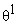,and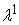) the value ofis calculated from the initial chosen point “O”and their respective values obtained in the base case “P”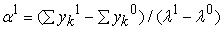(6)

For, the solution of the new system of equations formed by (3) and (4) will provide a new operation point (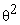,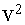and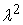) corresponding to the intersection of the solution trajectory (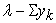curve) with the line whose new angular coefficient value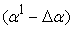was specified. For, the converged solution should provide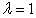.

By expanding (3) augmented by (4);(7)

in the Taylor series up to the first order terms, considering the preset value of, yields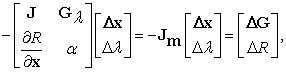(8)

where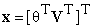, J is the Jacobian matrix, Jm is the modified Jacobian matrix andcorresponds to the partial derivatives of G with respect to. ΔG and ΔR denote the respective function mismatches of new system of equations. It should be noted that the mismatches would be equal to zero (or close to zero, e.g., below the adopted tolerance) for the base case PF solution. Thus, only ΔR will be different from zero due to the change in, e.g., due to itsdecrease. To obtain the lower part of thecurve, is considered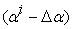.

Figure 1. Performance of the method, (a) λ-∑yk curve, (b) region around MLP enlarged
3.1. Flowchart of the Method for the Tracing of P-V Curve

For a better understanding of the general procedure for changing the set of lines, it was defined a flowchart as shown in Figure 2. Figure 1 is also used to illustrate the procedure shown in the flowchart, Figure 1(a) is shown all tracing ofcurve using the flowchart of Figure 2. In Figure 1(b) shows the region around MLP enlarged showing the set of line that belongs to midpoint (MP).

### 4. Results

This Section discusses the results of applying of the method to the ill-conditioned system (11 buses) and the IEEE system (300 buses). For all simulations the power mismatch convergence threshold was 10–4 p.u., and the values adopted for maximum number of iteration was 10, respectively. Upper and lower tap limits of, respectively, 1.05 and 0.95 were adopted. The loads were modeled as constant power and the parameter λ was used to simulate the real and reactive load increment, considering a constant power factor. The increase in load was followed by a generation increase using λ. The tap adjustment in the on load-tap changers (OLTC) transformers consisted of including the tap position as a dependent variable, whereas the controlled bus voltage magnitude was considered as an independent variable. The method of accounting for Q-limit at PV buses and tap limits is the same as in conventional PF methods. At every iteration the reactive generations at all PV buses are compared to their respective limits. In case of violation, a PV bus is switched to type PQ. This bus can be switched back to PV in future iterations. Tap limit violations are also checked.

4.1. Performance of the Method for the 11 Bus System

This case is reported as ill-conditioned  since it was found divergent with the Newton method. The system contains 11 buses and 11 branches, as shown in Table 1 and Table 2. The Figure 1 shows the diagram of the 11 bus system.

#### Table 2. Data of the branches

Figure 4. Performance of the method for the 11 bus system with Δα = 1.0: (a) ∑yk in function of λ (proposed method), (b) P-V curves (stored points), (c) number of iterations

The Figure 4 shows the results obtained for an ill-conditioned system of 11 buses, the same system shown in . All the taps of the system were changed to the nominal position, i.e., equal to 1 p.u. The step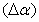adopted for the angular coefficient of the set of lines located in the planewas kept fixed during the tracing of the P-V curve,, Figure 4(a). The angular coefficient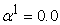, because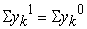in the “flat start. A Figure 4(b) shows the respective points (red points) of the critical bus (V11) stored by the method during the obtaining of thecurve of Figure 4(a). The number of iterations can be seen in Figure 4(c).

In the Figure 5 presents the results of the same system of 11 buses with step reduction, i.e.,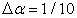. A Figure 5(b) shows the respective points (red points) of the critical bus (V11) and of the bus 8 (blue points) stored by the method during the obtaining of thecurve. The Figure 5(c) presents the number of iterations. The value of the MLP of critical bus was (λ, V11) = (0.69949, 0.56639 p.u.) with reduction step and with step equal, the value was (λ, V11) = (0.6896, 0.64428 p.u.), what is to be expected. The point “P”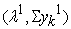to the “flat start was obtained with 3 iterations.

Figure 5. Performance of the method for the 11 bus system with step reduction, Δα = 0.1: (a) ∑yk in function of λ (proposed method), (b) P-V curves (stored points), (c) number of iterations
4.2. Performance of the Method for Large Systems

For large systems, whose thecurves presents characteristic as of the IEEE-300 bus system, seen in Figure 6, and whose the complete flowchart is used, the method shows the additional advantage of computing a larger number of operating points around the MLP. This occurs naturally due to the proximity of the MP coordinates with those of the MLP. Therefore, despite using the same step size during the whole curve tracing, the method automatically provides a larger number of points in the MLP region. This fact is interesting for systems with these characteristics because it indirectly enables a more precise calculation of the value of the loading margin without requiring a special strategy for the reduction of the step size.For these systems, whose thecurves exhibit a behavior similar the parables, the method remained restricted until the point * of the flowchart.

Figure 6. Performance of the method for the IEEE-300 bus system with Δα = 1.0 fixed: (a) ∑yk in function of λ (proposed method), (b) details of the tracing process of the MLP region, (c) P-V curve of the critical bus (stored points), (d) number of iterations

The Figure 6(a) presents the obtaining of thecurve. The stepadopted for the angular coefficient of the set of lines located in the planewas kept fixed during the tracing of the all P-V curve,. The angular coefficient, becausein the “flat start. The point “P”to the “flat start was obtained with 3 iterations, details of the tracing process of the MLP region can be seen in Figure 6(b). The Figure 6(c) shows the respective points (red points) of the critical bus (V526) stored by the method during the obtaining of thecurve of Figure 6(a). The value of the MLP of critical bus was (λ, V526) = (1.05528, 0.72855 p.u.). The number of iterations can be seen in Figure 6(b).

Figure 7. Performance of the method for the IEEE-300 bus system with duplicate step, Δα = 2.0 fixed: (a) P-V curve of the critical bus (stored points) with duplicate step, (b) number of iterations
Figure 8. Performance of the method for post-contingency of the 638-bus system corresponding to part of South-Southeast Brazilian system with Δα = 1.0 fixed: (a) ∑yk in function of λ (proposed method), (b). details of the tracing process of the MLP region

The Figure 7(a) shows the performance of the method for the IEEE-300 bus system with duplicate step,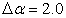fixed, P-V curve of the critical bus (stored points) with duplicate step is presented. The value of the MLP was (λ, V526) = (1.05709, 0.72699 p.u.) and the number of iterations can be seen in Figure 7(b).

Figure 8 shows the 638-bus system with the outage of the transmission line between the buses 147 and 149. In Figure 8(a) shows the tracing of thecurve of post-contingency of the system. Figure 8(b) shows details of the tracing process of the MLP region. The number of iterations can be seen in Figure 8(c).

### 5. Conclusion

As a priori, does not know the system to be studied and most ill-conditioned systems have loading margin lower than base case and the Newton method fail to obtain the point “P”, it was decided to start the method from default values “flat start” (all voltages in load buses initialized with unitary module and zero phase angle). To all tests realized the method obtained the “P” point and the complete tracing of the P-V curve without numerical problems related the Jacobian matrix (J).

Another important factor is related to contingency in the system. Very often a severe contingency leads to a contraction of the P-V curve with negative loading margins. With this methodology it is possible to obtain the post-contingency curve of these systems.

In terms of performance, the change of set of lines coordinates to the midpoint causes an automatic step size control around the MLP.

The results obtained with the technique to ill-conditioned systems (11 buses), IEEE test systems (300 buses) and the 638-bus system corresponding to part of South-Southeast Brazilian system, improved the characteristics of the conventional method and extended the convergence region around the singularity.

### Acknowledgement

The authors are grateful to the financial support provided by CNPq (Brazilian Research Funding Agencies) and UNESP campus of Tupã.

### References

  National System Operator (NSO). Plano Anual da Operação Energética – PEN 2011: relatório executivo. Rio de Janeiro, 2012. Disponível em: . Acesso em: 13 jun. 2014.In article  Taylor, C. W. Power system voltage stability. New York: McGraw-Hill, 1994.In article  Matarucco R. R., Bonini Neto A., Alves D. A. Assessment of branch outage contingencies using the continuation method. International Journal of Electrical Power & Energy Systems, v. 55, p. 74-81, 2014.In article CrossRef  Bonini Neto A., Alves D. A.. Improved Geometric Parameterization Techniques for Continuation Power Flow. IET Generation, Transmission & Distribution, v. 4, p. 1349-1359, 2010.In article CrossRef  Braz L. M. C., Castro C. A., Murari C. A. F. A critical evaluation of step size optimization based load flow methods. IEEE Transactions on Power Systems, 15(1): 202-207, 2000.In article CrossRef  Iwamoto S., Tamura Y.. A load flow calculation method for ill-conditioned power systems. IEEE Transactions on Power Apparatus and Systems, PAS-100 (4): 1736-1743, 1981.In article CrossRef  Meireles E. D. Uma contribuição ao estudo do problema de mal condicionamento de redes elétricas de potência sob o ponto de vista de estabilidade de tensão. Dissertação de Mestrado, FEEC/UNICAMP, Maio., 2005.In article  Wang Y., da Silva L.C.P., Xu W., Zhang Y. Analysis of ill-conditioned power flow problems using voltage stability methodology. IEE Proceedings on Generation, Transmission and Distribution, 148(5): 384-390, 2001In article CrossRef  Golub, G.H. and Van Loan, C.F.: Matrix Computations (Johns Hopkins University Press, 1983), 1st edn.In article  WSCC, Final Report, Voltage Stability Criteria, Undervoltage Load Shedding Strategy, and Reactive Power Reserve Monitoring Methodology, Reactive Power Reserve Work Group (RRWG), 1998, 154p.In article  Chiang, H. D.; Li, H.; Yoshida, H.; Fukuyama, Y., Nakanishi, Y. The generation of ZIP-V Curves for Tracing Power System Steady State Stationary Behavior Due to Load and Generation Variations. IEEE PES Summer Meeting, Edmonton, Alberta, Canada, 1999, p. 647-651.In article  R. Seydel, “From Equilibrium to Chaos: Practical Bifurcation and Stability Analysis”, 2ª ed., New York: Springer-Verlag, 1994, p. 407.In article  Bonini Neto A., and Alves D. A. “Studies of Contingencies in Power Systems through a Geometric Parameterization Technique, Part I: Mathematical Modeling.” World Journal Control Science and Engineering 2.1 (2014): 18-24.In article  Bonini Neto A., and Alves D. A. “Studies of Contingencies in Power Systems through a Geometric Parameterization Technique, Part II: Performance Evaluation.” World Journal Control Science and Engineering 2.1 (2014): 25-34.In article  Ajjarapu V. and Christy C., “The continuation power flow: A tool for steady state voltage stability analysis,” IEEE Trans. on Power Systems, vol. 7, pp. 416-423, Feb. 1992.In article CrossRef  Iba, K.; Suzuki, H.; Egawa, M.; Watanabe, T. Calculation of Critical Loading Condition with Nose Curve Using Homotopy Continuation Method. IEEE Trans. on Power Systems, v.6, n.2, 1991, p. 585-593.In article CrossRef  Chiang H. D., Flueck A. J., Shah K. S., and Balu N., “CPFLOW: A practical tool for tracing power systems steady-state stationary behavior due to load and generation variations,” IEEE Trans. Power Systems, vol. 10, pp. 623-633, May 1995.In article CrossRef  Garbelini E., Alves D. A., Bonini Neto A., Righeto E., Silva L. C. P., Castro C. A. An Efficient Geometric Parameterization Technique for the Continuation Power Flow. Electric Power Systems Research, v. 77, p. 71-82, EUA, 2007.In article  Alves D. A., da Silva L. C. P., Castro C. A., and da Costa V. F. “Continuation load flow method parameterized by power losses,” in Proceedings of the IEEE Power Engineering Society Winter Meeting (PES ’00), pp. 1123-1128, Singapore, January, 2000.In article  Zhao J. and Zhang B. “Reasons and countermeasures for computation failures of continuation power flow,” in Proceedings of the IEEE Power Engineering Society General Meeting (PES ’06), June 2006.In article  Tripathy S. C., Prasad G. D., Malik O. P. and Hope G. S.: Load-Flow solutions for ill-conditioned power systems by a Newton-like method, IEEE Trans. Power Appar. Syst., 1982, 101, (10), pp. 3648-3657.In article CrossRef